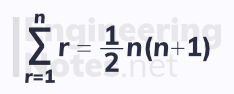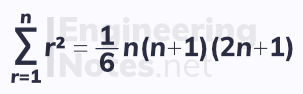top of page

#### Notes by Category University Engineering

Rate these notesNot a fanNot so goodGoodVery goodBrillRate these notes

# Series

Sigma notation is used to write series quickly. This describes each term of a series as an equation, where substituting in values for r gives that term in the series.

There are standard sums of series formulae that are used all the time:

### The Sum of Natural Numbers

To find the sum of a series of constant terms, use the formula:To find the sum of the first n natural numbers (positive integers):If the series does not start at r = 1, but at r = k, subtract one series from another, where the first goes from r = 1 to r = k, and the second from r = 1 to r = (k-1):Expressions in sigma notation can be rearranged to simplify complicated series:### The Sum of Squares

The first sum formula above, for constant terms, is linear. The second, for the sum of natural numbers, is quadratic. Therefore, the formula for the sum of square terms is cubic.

To find the sum of the sum of the squares of the first n natural numbers:### The Sum of Cubics

Following through with this pattern, the formula to find the sum of the cubes of the first n natural numbers is quartic:## The Method of Differences

The method of differences is used when the expansion of a series leads to pairs of terms that cancel out, leaving only a few single terms behind.

When the general term, u₁, of a series can be expressed in the form f(r) - f(r+1), the method of differences applies.This means that:To solve problems with the method of differences

1. Write out the first few terms to see what cancels

2. Write out the last few terms to see what cancels

3. Add the left overs from the beginning and the end

Often, you will need to use partial fractions

### Example## The Maclaurin Series

We often work with first and second order derivatives, but why stop there? Functions that can be written as an infinite sum of terms in the form axⁿ can be differentiated infinite times.

We already know of a few such examples:

• the binomial expansion of 1 / (1-x) forms an infinite polynomial, 1 + x + x² + x³ + x⁴ + ...

• the binomial expansion of 1 / √(1+x) forms an infinite polynomial, 1 + ½ x - ⅟₈ x² + ⅟₁₆ x³ - ⅟₃₂ x⁴ + ...

• eˣ = 1 + x + ½ x² + ⅟₆ x³ + ⅟₂₄ x⁴ + ...

This happens when x = 0 is substituted into increasing orders of derivatives, which, in turn, increases the power of the polynomial.

This is only true if the function can be differentiated an infinite number of times, and if the series converges.

When the above criteria are satisfied and each derivative has a finite value, the Maclaurin series expansion is valid:There are many standard versions of the Maclaurin series expansion that are useful to know, as they crop up a great deal:See All
bottom of page